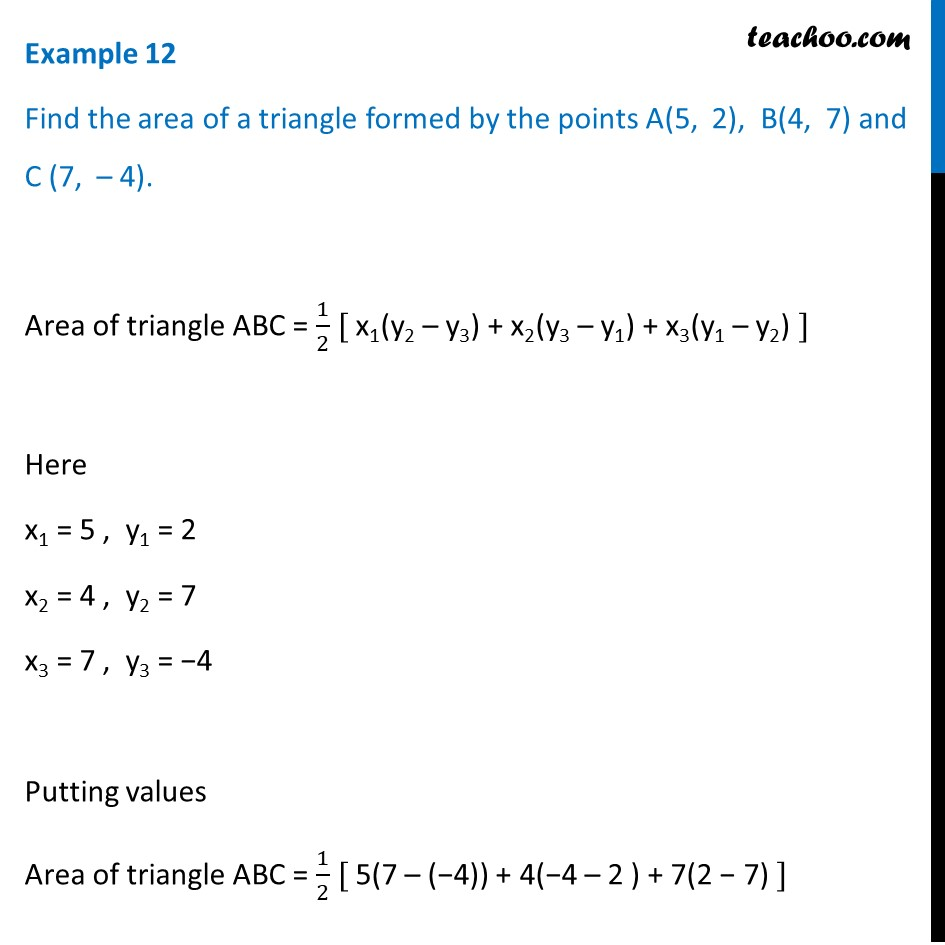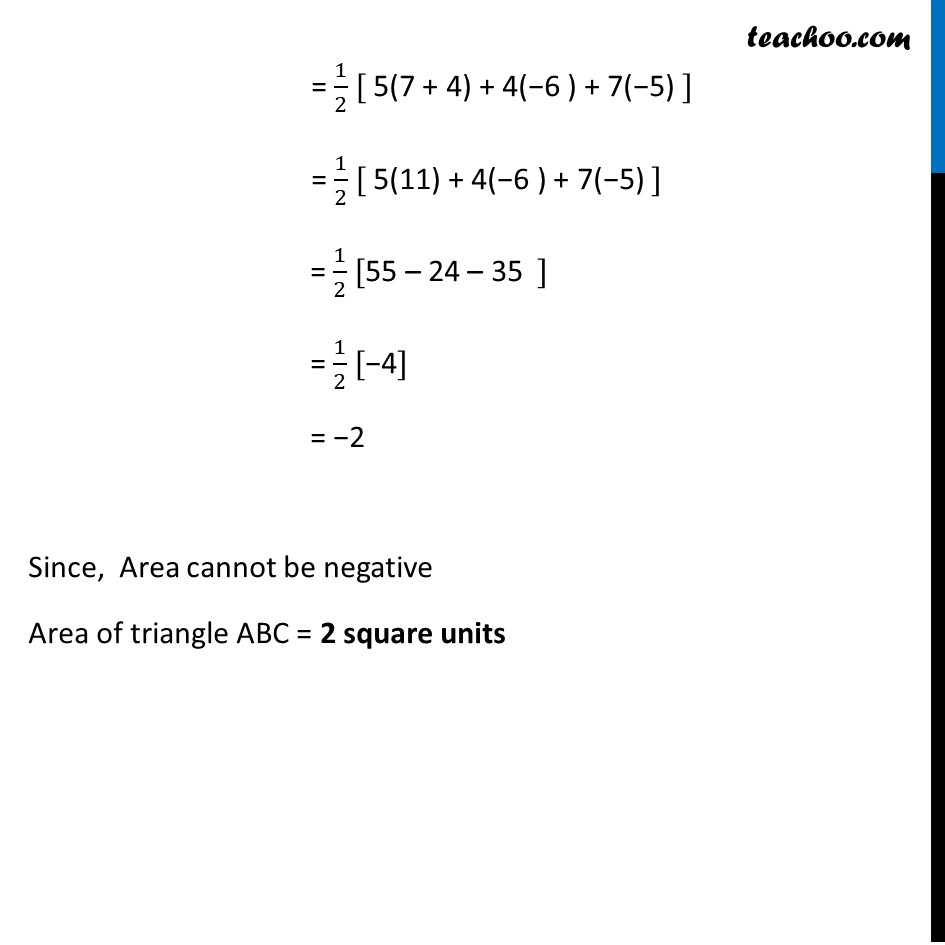Examples

Chapter 7 Class 10 Coordinate Geometry
Serial order wiseLearn in your speed, with individual attention - Teachoo Maths 1-on-1 Class

### Transcript

Question 2 Find the area of a triangle formed by the points A(5, 2), B(4, 7) and C (7, – 4). Area of triangle ABC = 1/2 [ x1(y2 – y3) + x2(y3 – y1) + x3(y1 – y2) ] Here x1 = 5 , y1 = 2 x2 = 4 , y2 = 7 x3 = 7 , y3 = −4 Putting values Area of triangle ABC = 1/2 [ 5(7 – (−4)) + 4(−4 – 2 ) + 7(2 − 7) ] = 1/2 [ 5(7 + 4) + 4(−6 ) + 7(−5) ] = 1/2 [ 5(11) + 4(−6 ) + 7(−5) ] = 1/2 [55 – 24 – 35 ] = 1/2 [−4] = −2 Since, Area cannot be negative Area of triangle ABC = 2 square units